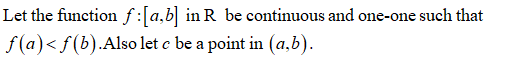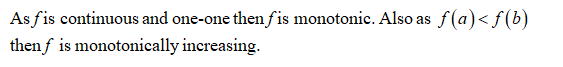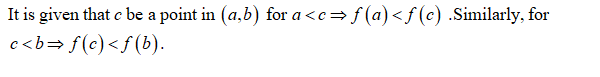# 13. Let the function f: [a, b] in R be continuous and one-to-one and such that f(a) < f(b). Let c be a point in the open interval (a, b). Prove that f(a) < f(c) < f(b).

Question
1 views

13. Let the function f: [a, b] in R be continuous and one-to-one and such that f(a) < f(b). Let c be a point in the open interval (a, b). Prove that f(a) < f(c) < f(b).

check_circle

Step 1Step 2Step 3...

### Want to see the full answer?

See Solution

#### Want to see this answer and more?

Solutions are written by subject experts who are available 24/7. Questions are typically answered within 1 hour.*

See Solution
*Response times may vary by subject and question.
Tagged in

### Math